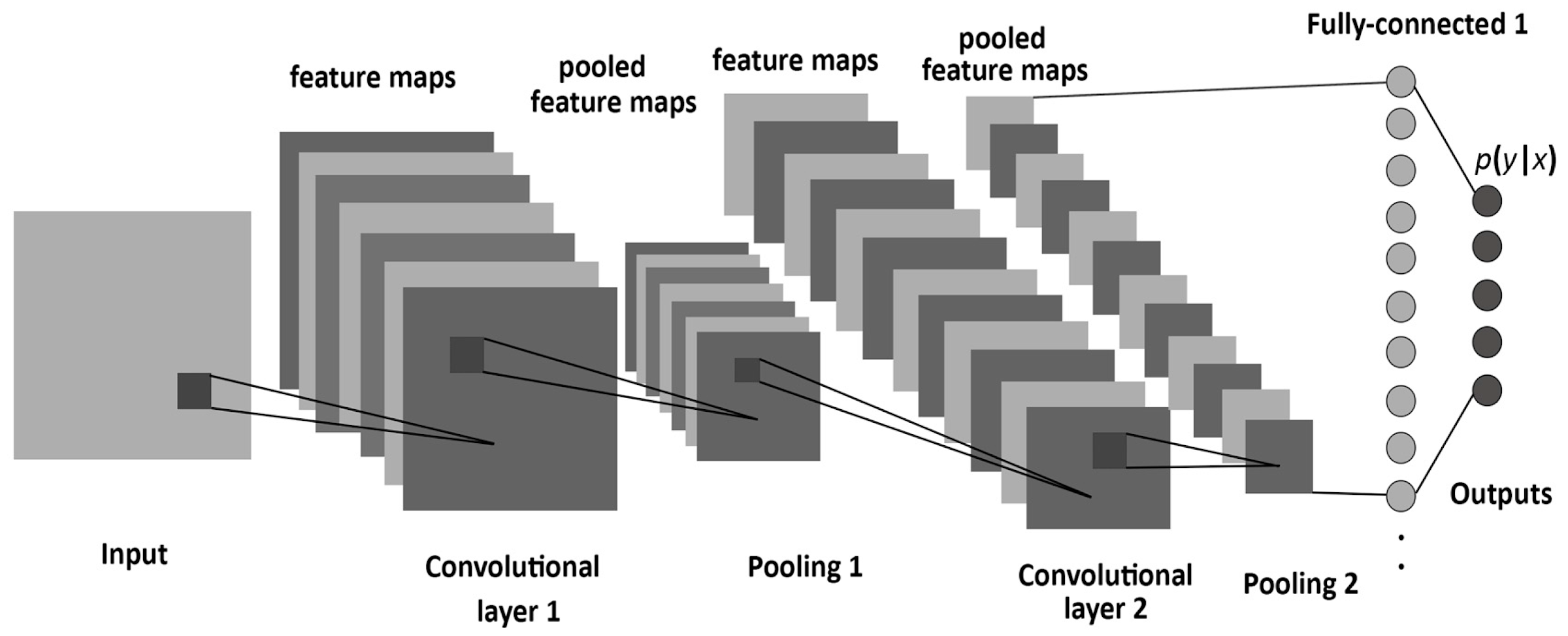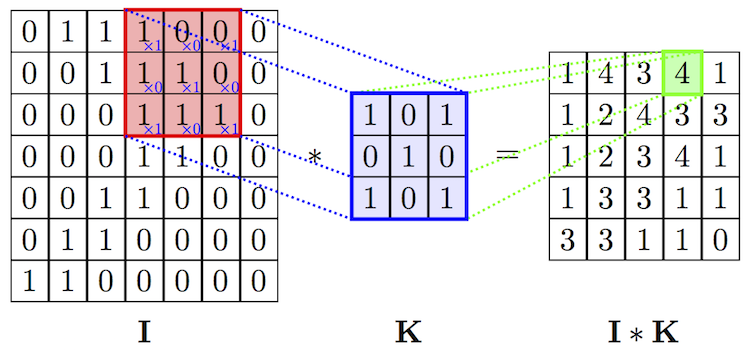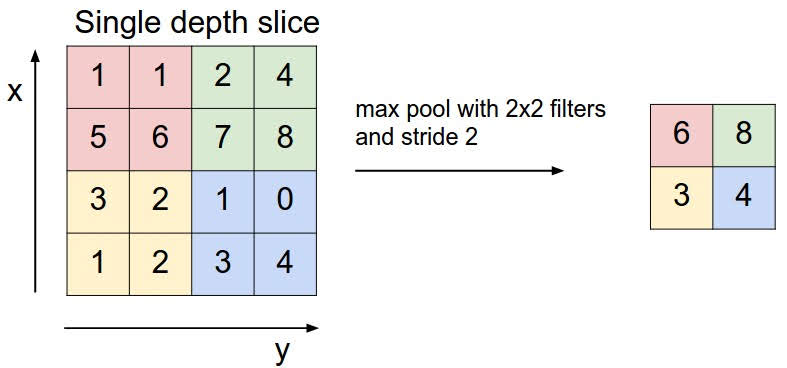# Intro to Convolutional Neural Network

Categories:

Updated:

Convolutional neural network (CNN) is mostly used in computer vision problems such as image classification, object detection, neural style transfer, and etc.

Typically, for computer vision problems, we use RGB of each pixel. If the image size is 10x10, the input shape is actually 10x10x3, as there are three color density values in each pixel(red, green, blue).

# Inspiration

Convolutional networks were inspired by biological processes in that the connectivity pattern between neurons resembles the organization of the animal visual cortex. Individual cortical neurons respond to stimuli only in a restricted region of the visual field known as the receptive field. The receptive fields of different neurons partially overlap such that they cover the entire visual field.

# Why CNN?

Although fully connected feedforward neural networks can be used to learn features as well as classify data, it is not practical to apply this architecture to images.

A very high number of neurons would be necessary, even in a shallow (opposite of deep) architecture, due to the very large input sizes associated with images, where each pixel is a relevant variable. For instance, a fully connected layer for a (small) image of size 100 x 100 has 10000 weights for each neuron in the second layer.

The convolution operation brings a solution to this problem as it reduces the number of free parameters, allowing the network to be deeper with fewer parameters. For instance, regardless of image size, tiling regions of size 5 x 5, each with the same shared weights, requires only 25 learnable parameters.

In conclusion by reducing number of neurons, CNN prevents overfitting and vanishing/exploding gradients. Also it simulates visual cortex so is suitable for computer vision problems.

# Architecture

Convolutional neural networks are usually made up of three types of layers.## 1. Convolutional

Convolutional layers apply a convolution operation to the input, passing the result to the next layer. Each convolutional neuron processes data only for its receptive field. The convolution emulates the response of an individual neuron to visual stimuli.## 2. Pooling

Convolutional networks may include pooling layers, which combine the outputs of neuron clusters at one layer into a single neuron in the next layer.

For example, max pooling uses the maximum value from each of a cluster of neurons at the prior layer. Another example is average pooling, which uses the average value from each of a cluster of neurons at the prior layer.## 3. Fully Connected

Usually, we add traditional multi-layer perceptron neural network (MLP) layers at the tail of the convolutional neural network. We call these layers fully-connected layers.

Categories: